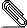# Re: [Hol-info] "Wrong" definitions of transcendental functions (sin, cos, exp)?

```Sorry... I'm wrong, these definitions are correct.  I was confused by
the definition of "suminf" for reals with the one for extreals.   The
one for reals is based on "sums" and "convergent", should be able to
handle negative values.```
```
So, it should be possible to prove a full version of EXP_LE_X.

--Chun

Il 18/03/19 20:05, Chun Tian (binghe) ha scritto:
> Hi,
>
> does anyone notice that, the 3 basic transcendental functions (sin, cos,
> exp) in HOL4 are only correctly defined on positive inputs?
>
> I say this because all these definitions are based on suminf which gives
> desired results only for all positive summations:
>
>    [exp]  Definition
>
>       ⊢ ∀x. exp x = suminf (λn. (λn. (&FACT n)⁻¹) n * x pow n)
>
>    [sin]  Definition
>
>    ⊢ ∀x.
>          sin x =
>          suminf
>            (λn.
>              (λn. if EVEN n then 0
>                   else -1 pow ((n − 1) DIV 2) / &FACT n) n * x pow n)
>
>    [cos]  Definition
>
>    ⊢ ∀x.
>          cos x =
>          suminf
>            (λn.
>              (λn. if EVEN n then -1 pow (n DIV 2) / &FACT n else 0) n *
>                 x pow n)
>
> where suminf is the sup of partial sums of first n items:
>
>> suminf;
> val it = ⊢ ∀f. suminf f = @s. f sums s: thm
>> sums;
> val it = ⊢ ∀f s. f sums s ⇔ (λn. sum (0,n) f) ⟶ s: thm
>
> by definition, exp x = 1 + x + x^2/2! + x^3/3! + ..., but if x < 0, the
> summation will be alternatively positive and negative, the "sup" should
> be the first item, i.e. with some efforts one should be able to prove:
> !x. x < 0 ==> (exp(x) = 1)
>
> --Chun
>
> ------------- below are some background story ----------------
>
> yesterday I started to need a small result of exponential function
> (exp), namely:
>
> |- !x. &0 <= x ==> (&1 - x) <= exp (-x)
>
> I noticed that, HOL Light has a theorem (REAL_EXP_LE_X) in its
> "Multivariate/transcendental.ml" saying:
>
> |- !x. &1 + x <= exp(x)
>
> this would be one I need (by instantiating `x` to `-x`) but in HOL4 I
> only see a restricted version (EXP_LE_X) with antecedents `0 <= x` in
> "src/real/transcScript.sml":
>
> |- !x. &0 <= x ==> (&1 + x) <= exp(x)
>
> Obviously this theorem (and the whole transcTheory) was ported from HOL
> Light's "Library/transc.ml" (REAL_EXP_LE_X), because all definitions and
> proofs are essentially identical.
>
> I can't easily port REAL_EXP_LE_X from HOL Light's
> "Multivariate/transcendental.ml", because the "exp" there was defined as
> the real part of complex-valued "exp". So I started to prove the full
> version of EXP_LE_X by myself.  My idea was to use the existing EXP_LE_X
> for the case "0 <= x", and the case `x < -1` is trivial, because `1 + x
> < 0` and `0 <= exp(x)`. Thus I only need to prove for the case `-1 <= x
> < 0`:
>
>    0 ≤ f x
>    ------------------------------------
>      0.  x < 0
>      1.  -1 < x
>      2.  Abbrev (f = (λx. exp x − (1 + x)))
>      3.  Abbrev (g = (λx. exp x − 1))
>      4.  ∀x. (f diffl g x) x
>      5.  ∀x. x ≤ 0 ⇒ g x ≤ 0
>      6.  f 0 = 0
>    : proof
>
> so far so good, I have proved that, the differentiation 'g' of f(x) =
> exp(x) - (1+x) is negative in [-1,0], and f(0) = 0. Then I was going to
> look into my calculus books to find proofs that f() is mono-decreasing
> in [-1,0] to finish the entire proof. Then, I suddenly found the sad
> news, that HOL4's "exp" function is not correctly defined on negative
> inputs.
>
>
>
>
>

```signature.asc
Description: OpenPGP digital signature

```_______________________________________________
hol-info mailing list
hol-info@lists.sourceforge.net
https://lists.sourceforge.net/lists/listinfo/hol-info
```